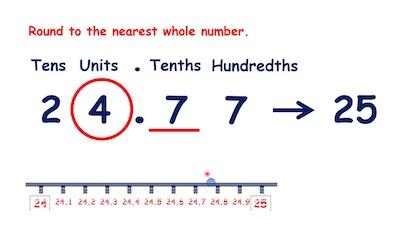# Rounding Off Whole Numbers

When you are looking to round off whole numbers, it’s important to keep in mind that the rounded number is an approximate value and not the real value. So, you need to ensure that you know this since it can affect your results.

Check out the best rounding calculators.

One of the things that you need to know when you are looking for rounding off whole numbers is that you need to take into consideration the main rules of rounding numbers.

## Rounding Numbers Rules

In case you don’t recall, for all numbers that end in 5 or higher, you will need to go up. On the other hand, when the last digit of your number ends up in a digit lower than 5, you’ll maintain it.

For example, 54 will be rounded to 50 while 55 will be rounded to 60.

## #1: Rounding Off A Number To The Nearest Tens Digit:

When you are looking to round off a number to the nearest tens digit, you will need to take a look at the number to the right of the tens digit of the rounding number.

Notice that the tens digit is the digit that is second from last in a number, before the ones digit. Let’s say you’re looking at the number 13. In this case, you need to look at number 3.

As we already explained to you above, if that number is less than 5, keep the rounding number the same; if it is greater than or equal to 5 round the rounding number up one digit. Here are some examples:

• 12 –> 10
• 114 –> 110
• 57 –> 60
• 1,334 –> 1330
• 1,488 –> 1490
• 97–> 100

Confirm the results on our nearest tens calculator.

## #2: Rounding Off A Number To The Nearest Hundreds Digit:

The truth is that the same rule applies here. The main difference is that instead of looking at the tens digit, you will need to look at the hundreds digit this time.

So, you’ll need to check out the hundreds digit, which is the third from last in a number, just before the tens digit. For example, in the number 1,234, the 2 is in the hundreds digit.

Then, you will need to use the number to the right of the hundreds digit, the tens digit, to see if you should round that number up or down, making the numbers after it even 00s. Here are some examples:

• 7,891 — > 7,900
• 15,753 –> 15,800
• 99,961 –> 100,000
• 3,350 –> 3,400
• 450 –> 500

## #3: Rounding Off A Number To The Nearest Thousands Digit:

Again, the same rules apply here. However, now you need to locate the thousands digit, which is fourth from the end of a number, and then check out the digit in the hundreds place, which will be to the right of that number.

Consider checking out our nearest thousand calculator.

If the digit is less than 5, round down, and if it’s greater than or equal to 5, round up. Here are a few more examples to consider:

• 8,800 –> 9,000
• 1,015 –> 1,000
• 12,450 –> 12,000
• 333,878 –> 334,000
• 400,400 –> 400,000